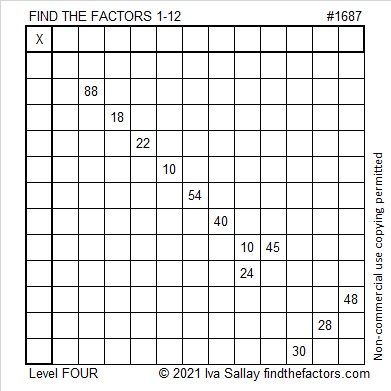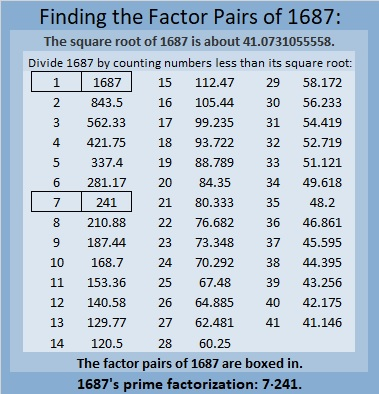# 1687 Fly Me to the Moon!

Contents

### Today’s Puzzle:

A witch flying on a broomstick in front of a bright full moon is a common Halloween image. Here is a level 4 puzzle shaped like a broom. If you succeed in solving it, you might just feel like you are flying to the moon, too. Just write the numbers from 1 to 12 in both the first column and in the top row so that those numbers and the given clues form a multiplication table. Best Witches!### Factors of 1687:

1687 is divisible by 7 because 16 is the double of 8, and the last digit is 7.
217, 427, 637, 847, 1057, 1267, 1477, 1687, 1897 are all divisible by 7.

• 1687 is a composite number.
• Prime factorization: 1687 = 7 × 241.
• 1687 has no exponents greater than 1 in its prime factorization, so √1687 cannot be simplified.
• The exponents in the prime factorization are 1 and 1. Adding one to each exponent and multiplying we get (1 + 1)(1 + 1) = 2 × 2 = 4. Therefore 1687 has exactly 4 factors.
• The factors of 1687 are outlined with their factor pair partners in the graphic below.### More About the Number 1687:

1687 is the hypotenuse of a Pythagorean triple:
840-1463-1687, which is 7 times (120-209-241).

This site uses Akismet to reduce spam. Learn how your comment data is processed.